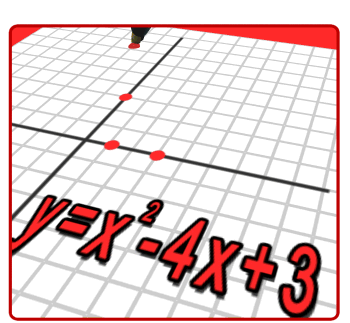# Algebra

•Unit A:   Linear Equations, Systems of Equations and Arithmetic Sequences

Unit B:   Linear Inequalities and Systems of Inequalities

Unit C:   Functions and Volume

Unit D:   Associations in Data

Unit E:   Pythagorean Theorem and Irrational Numbers

Unit F:   Exponents

Unit G:   Exponential Functions and Geometric Sequences

Unit H:  Polynomials